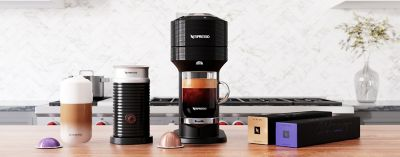# Nespresso## Nespresso

Showing 1 - 48 of 48 products

## Nespresso

Showing 1 - 48 of 48 products
Earn 3x points
Earn 3x points
Best Seller
Earn 3x points
Earn 3x points
Earn 3x points
Earn 3x points
Earn 3x points
Earn 3x points
Earn 3x points
Earn 3x points
Earn 3x points
Earn 3x points
Earn 3x points
Earn 3x points
Earn 3x points
Earn 3x points
Earn 3x points
Earn 3x points
Earn 3x points
Earn 3x points
Earn 3x points
Earn 3x points
Earn 3x points
Earn 3x points
Earn 3x points
Earn 3x points
Earn 3x points
Earn 3x points
Earn 3x points
Earn 3x points
Earn 3x points
Earn 3x points
Earn 3x points